6187

Chemistry Inorganic Chemistry Level: Misc Level

An ion and its parent atom have the same

a.electron configuration

b. atomic number

c. number of charges

d. chemical reactivity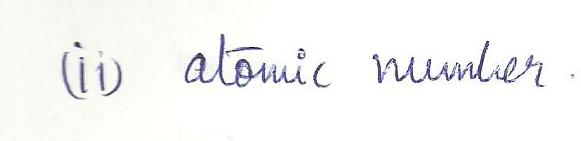5347

Chemistry Inorganic Chemistry Level: Misc Level

Arrange the following in order of increasing melting points.Cs, BaCI, Cdiamond , H2, HF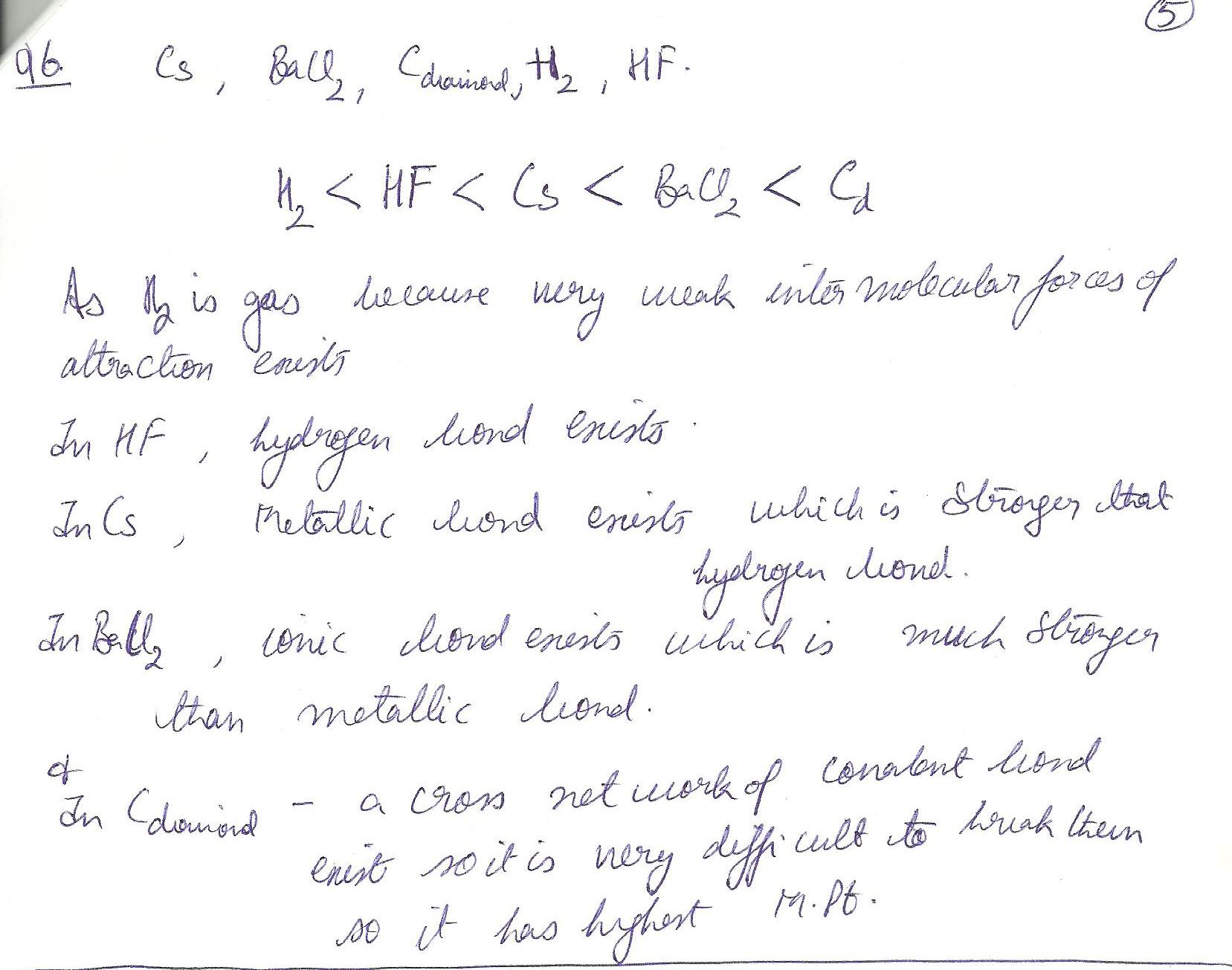5344

Chemistry Inorganic Chemistry Level: Misc Level

A mixture of Ar CH4,H2, CO, and PCL5 is released in back of the classroom. Which gas reaches the front of the classroom first?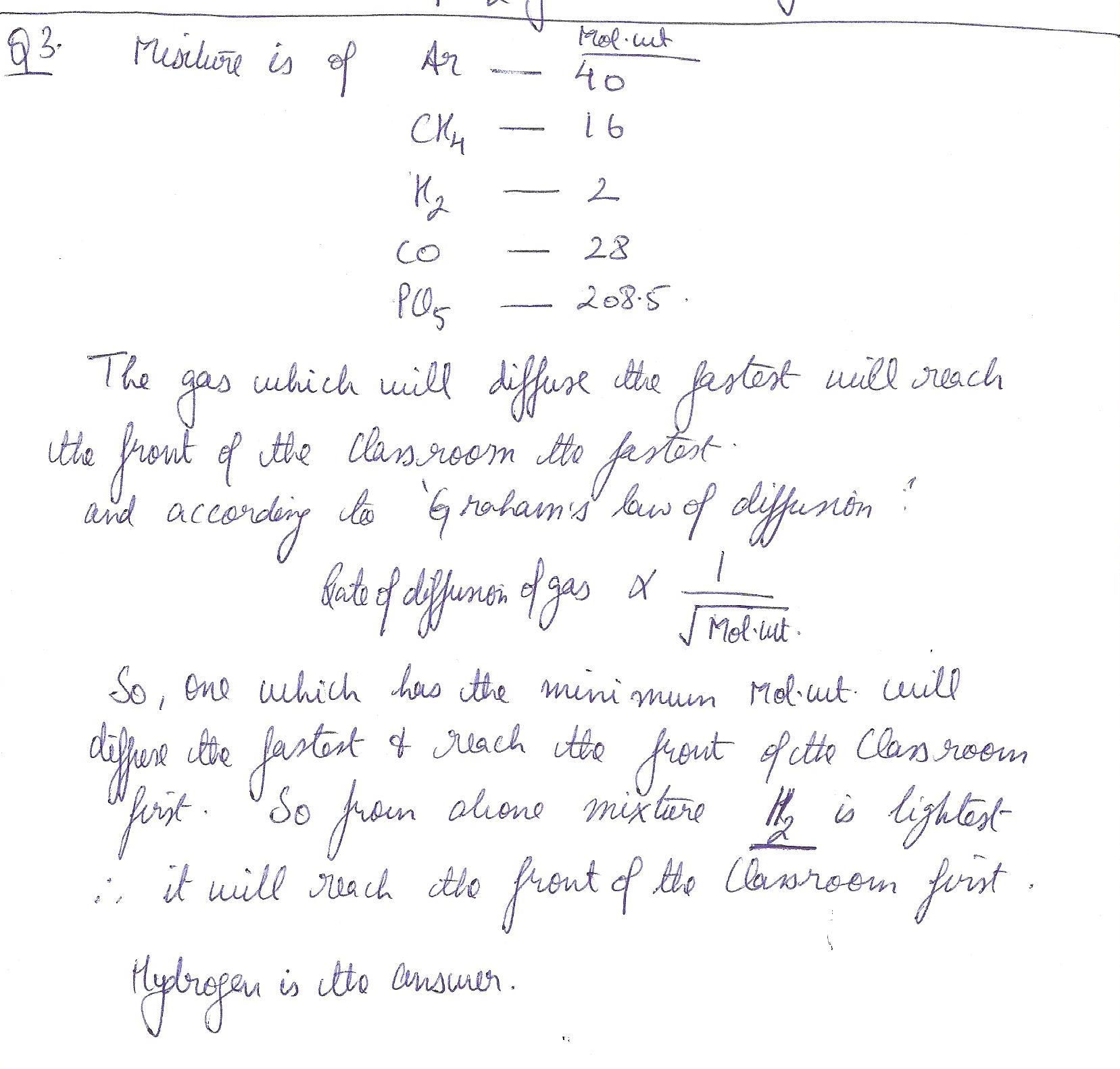4226

Chemistry Inorganic Chemistry Level: Misc Level

Give the pH of a solution that is 1000 times more basic than a solution with a pH of 9.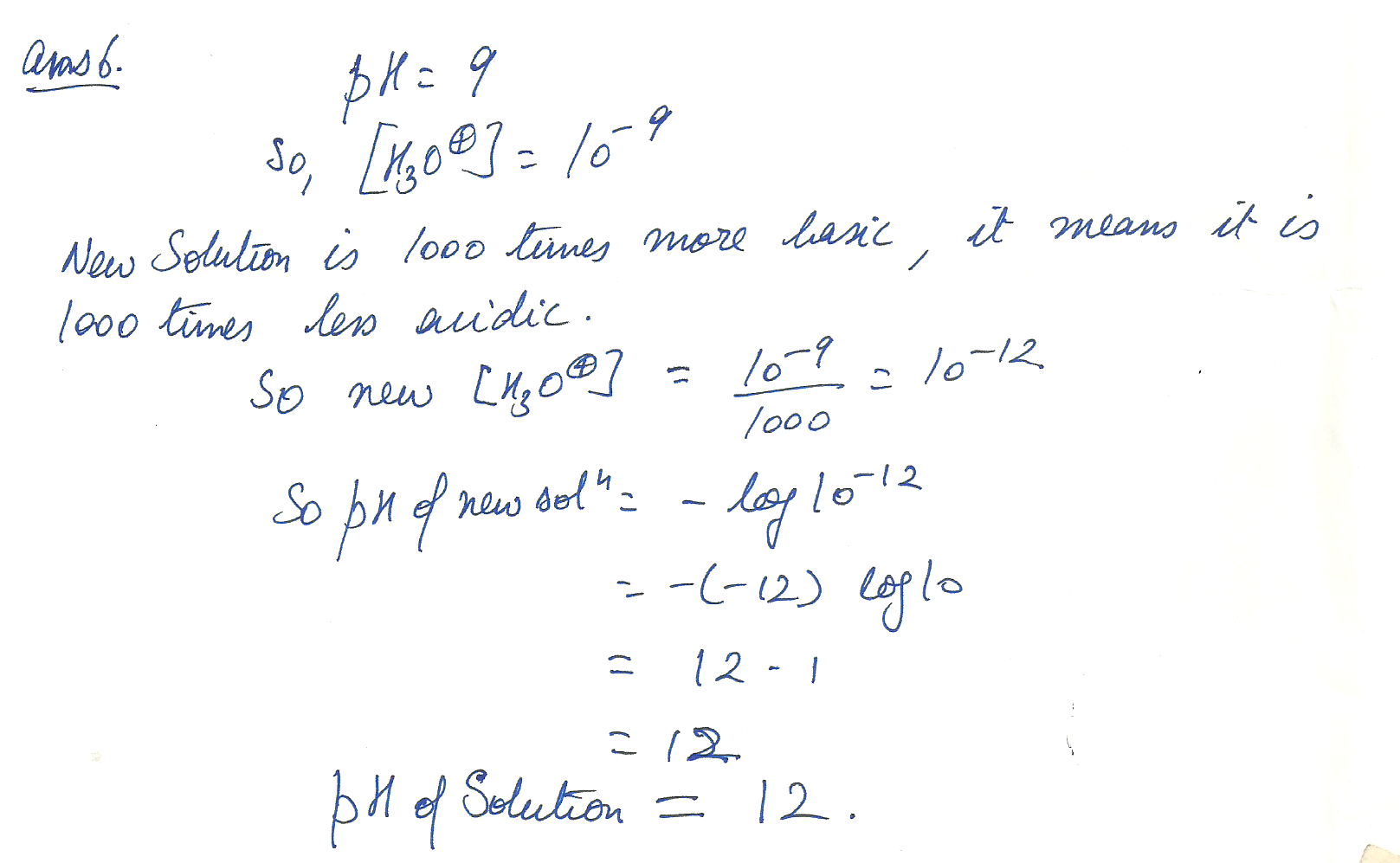4225

Chemistry Inorganic Chemistry Level: Misc Level

Give the pH of a solution that is 100 times less acidic than a solution with a pH of 3.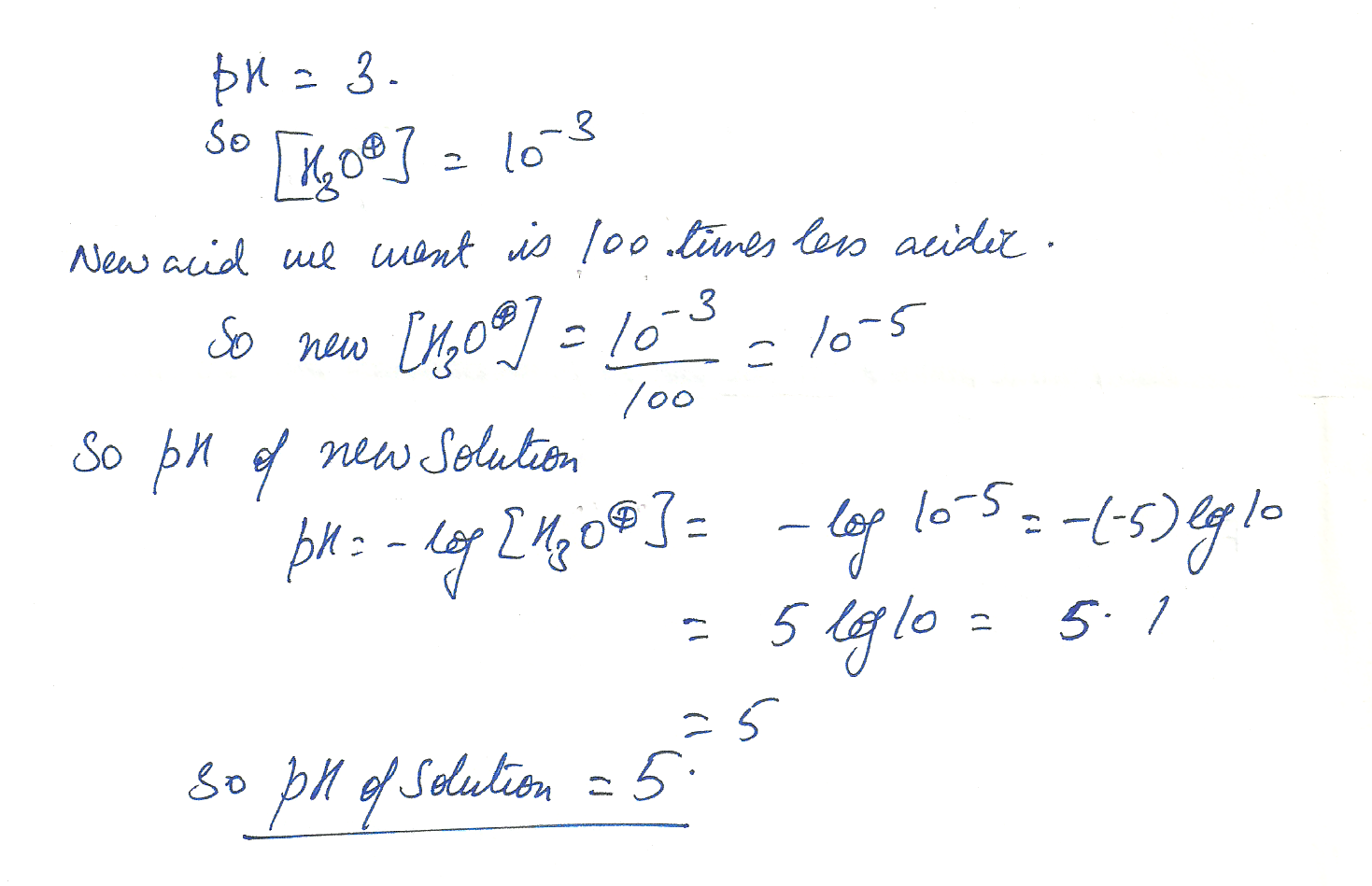4224

Chemistry Inorganic Chemistry Level: Misc Level

Identify the acid, base, conjugate acid, and conjugate base in the following H2S +NH3 <-------> NH4 +HS-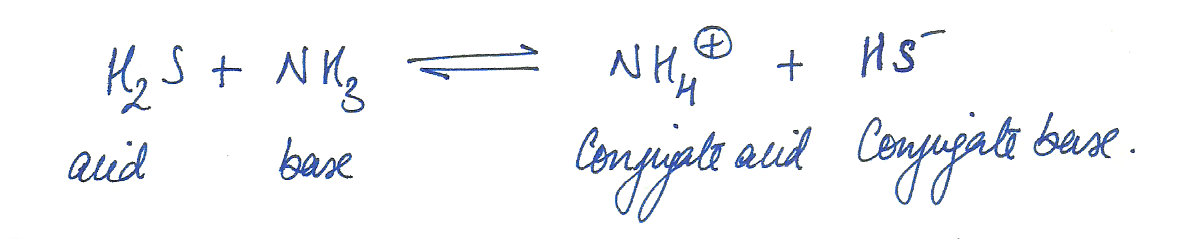4223

Chemistry Inorganic Chemistry Level: Misc Level

Write the chemical reaction that describes the reaction of the weak acid HNO2 with water. Identify the acid, base, conjugate acid, and conjugate base in your reaction.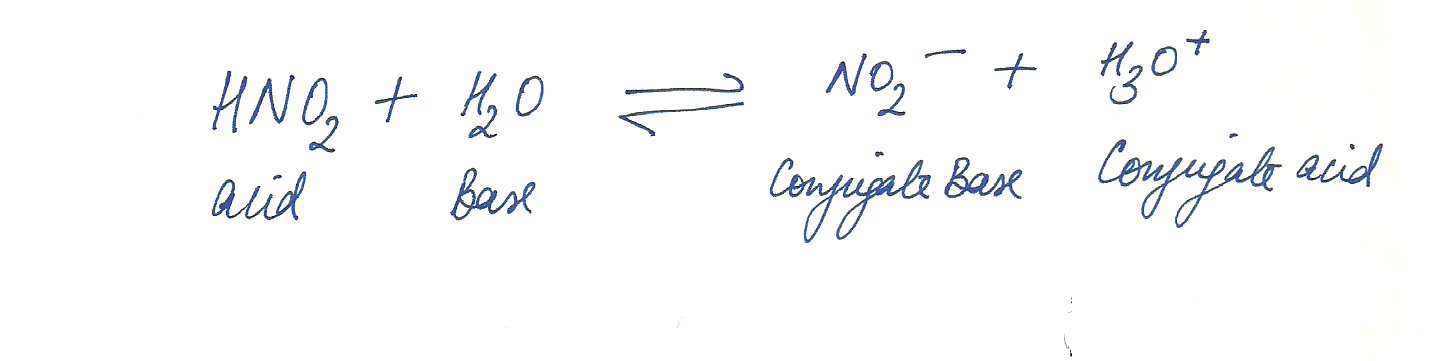4222

Chemistry Inorganic Chemistry Level: Misc Level

Give the pH, (OH- ) and indicate acid, base, or neutral for a solution that has (H3O+) of 1x10-11. ( 10 with a little minus sign and little 11 to the upper right of the 10. )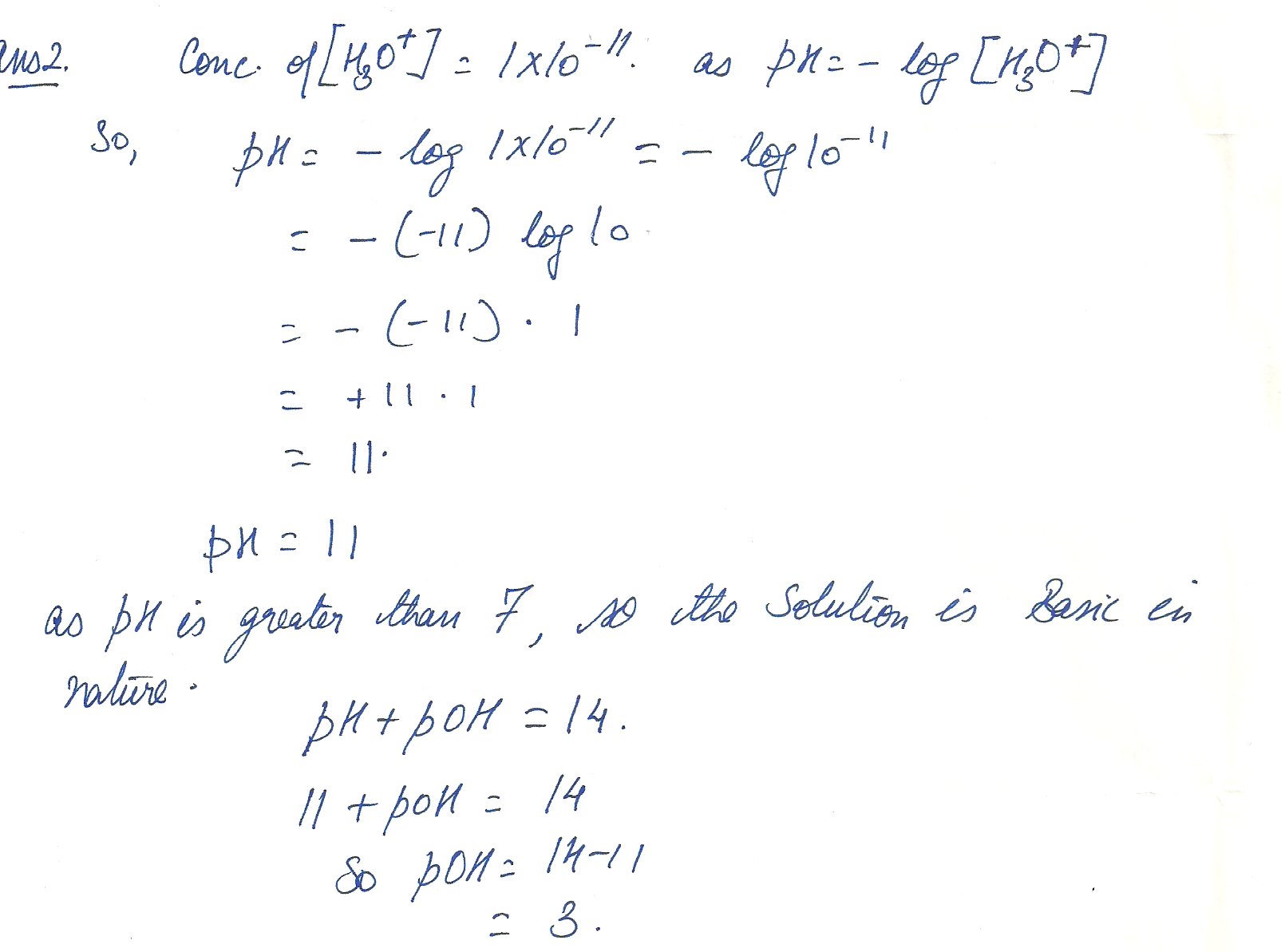4184

Chemistry Inorganic Chemistry Level: Misc Level

What Volume of 0.200 M HCI solution is needed to exactly neutralize 25.0 ML of 0.150 M NaOH solution?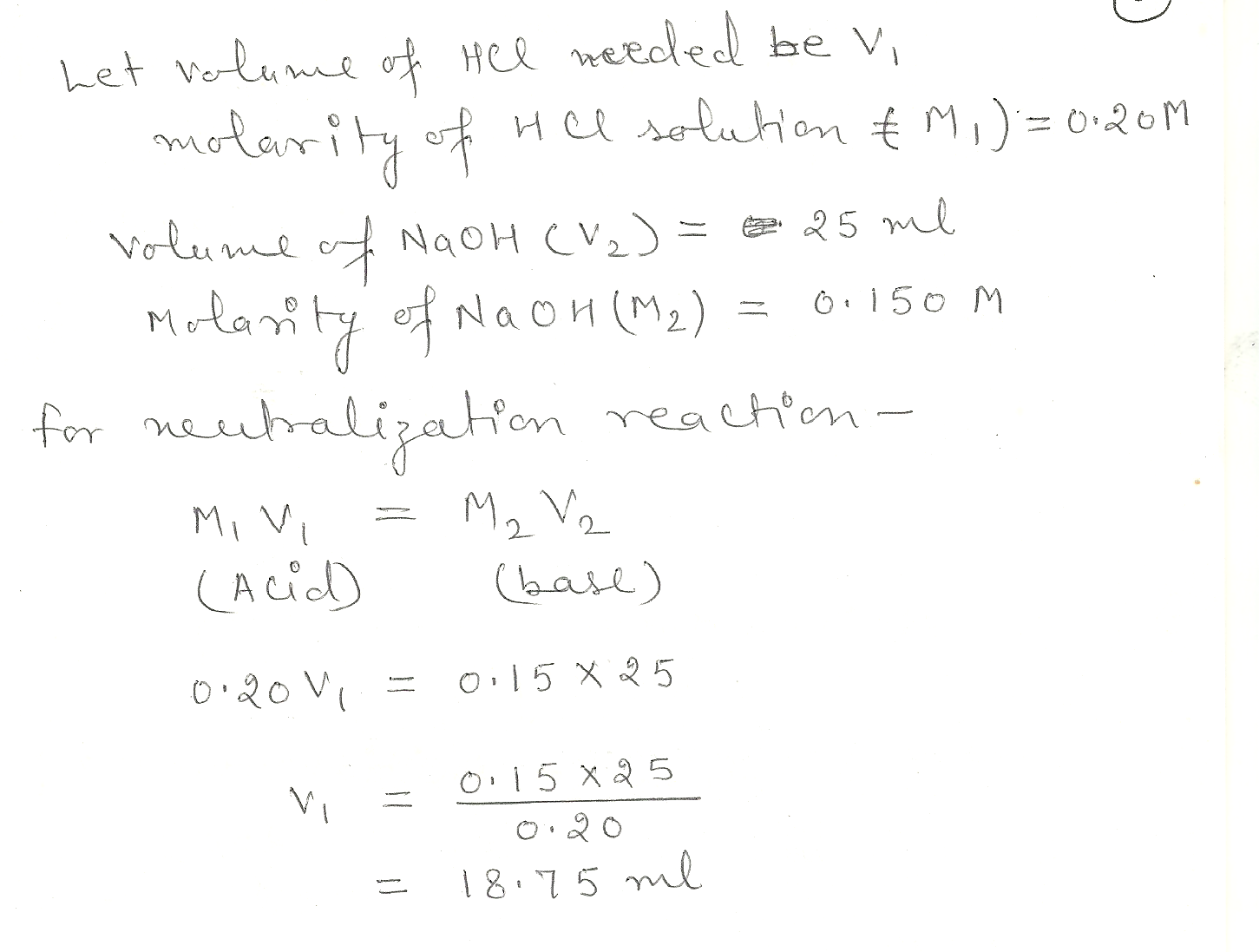4183

Chemistry Inorganic Chemistry Level: Misc Level

Calculate the new concentraion (molarity) that results when 250. ML of water is added to 125 MLof 0.251 M HCI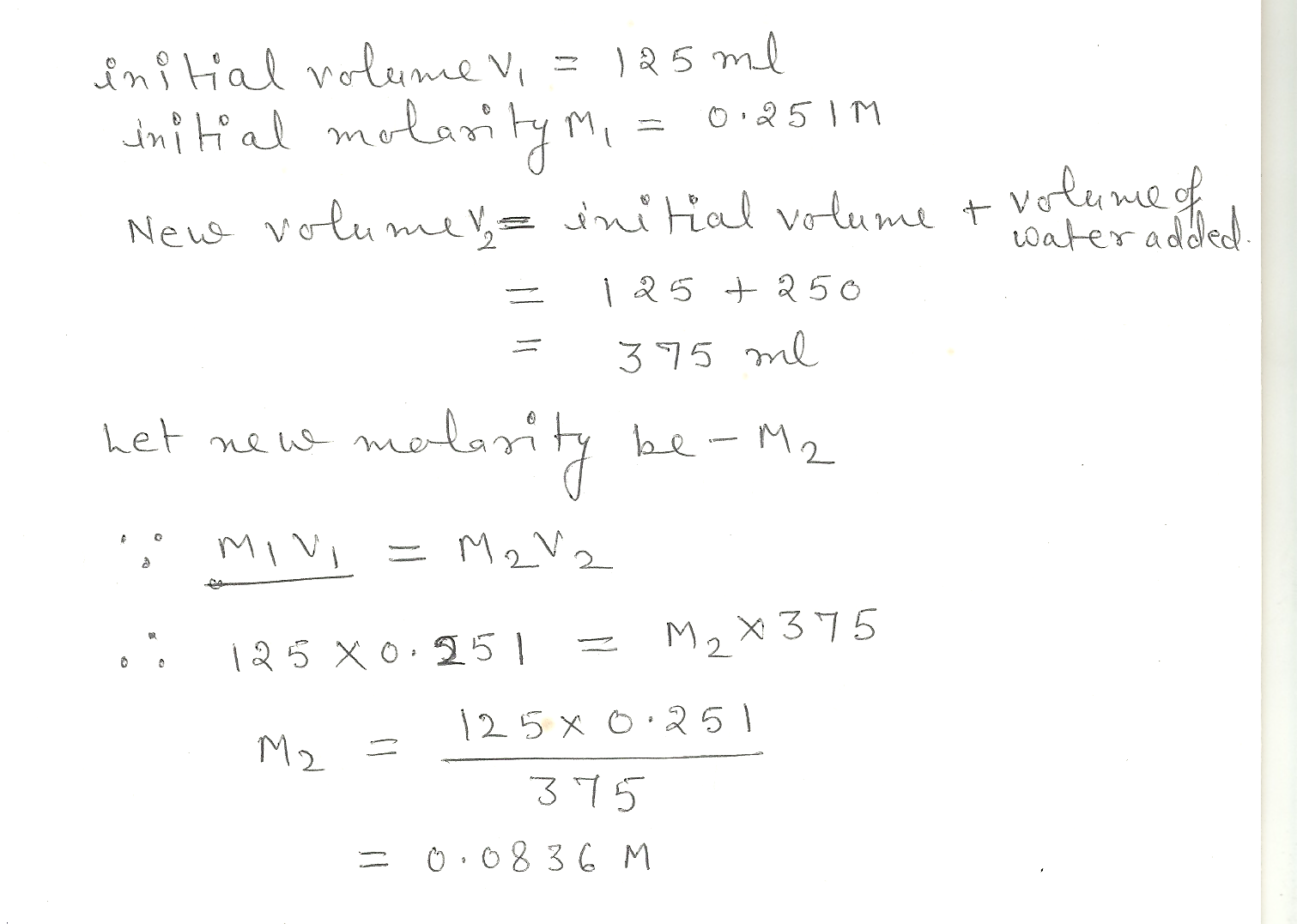4181

Chemistry Inorganic Chemistry Level: Misc Level

Calculate the number of moles and number of grams of HCI contained in 1.29 L of 0.411 M H CI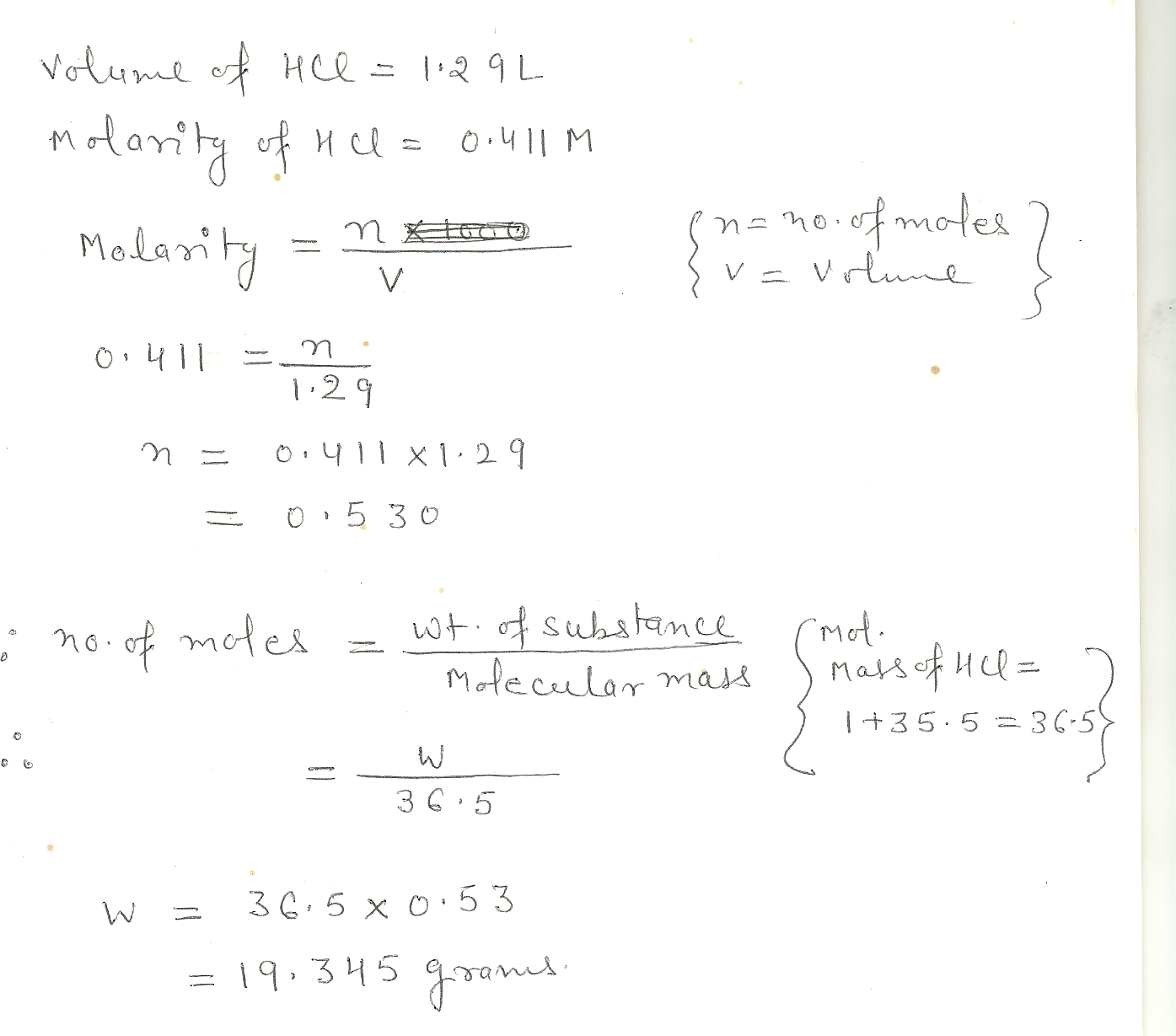4180

Chemistry Inorganic Chemistry Level: Misc Level

How many grams of sugar are contained in 250. g of an aqueous mixture that contains (by mass) 10.% sugar and 5.0% alcohol. ?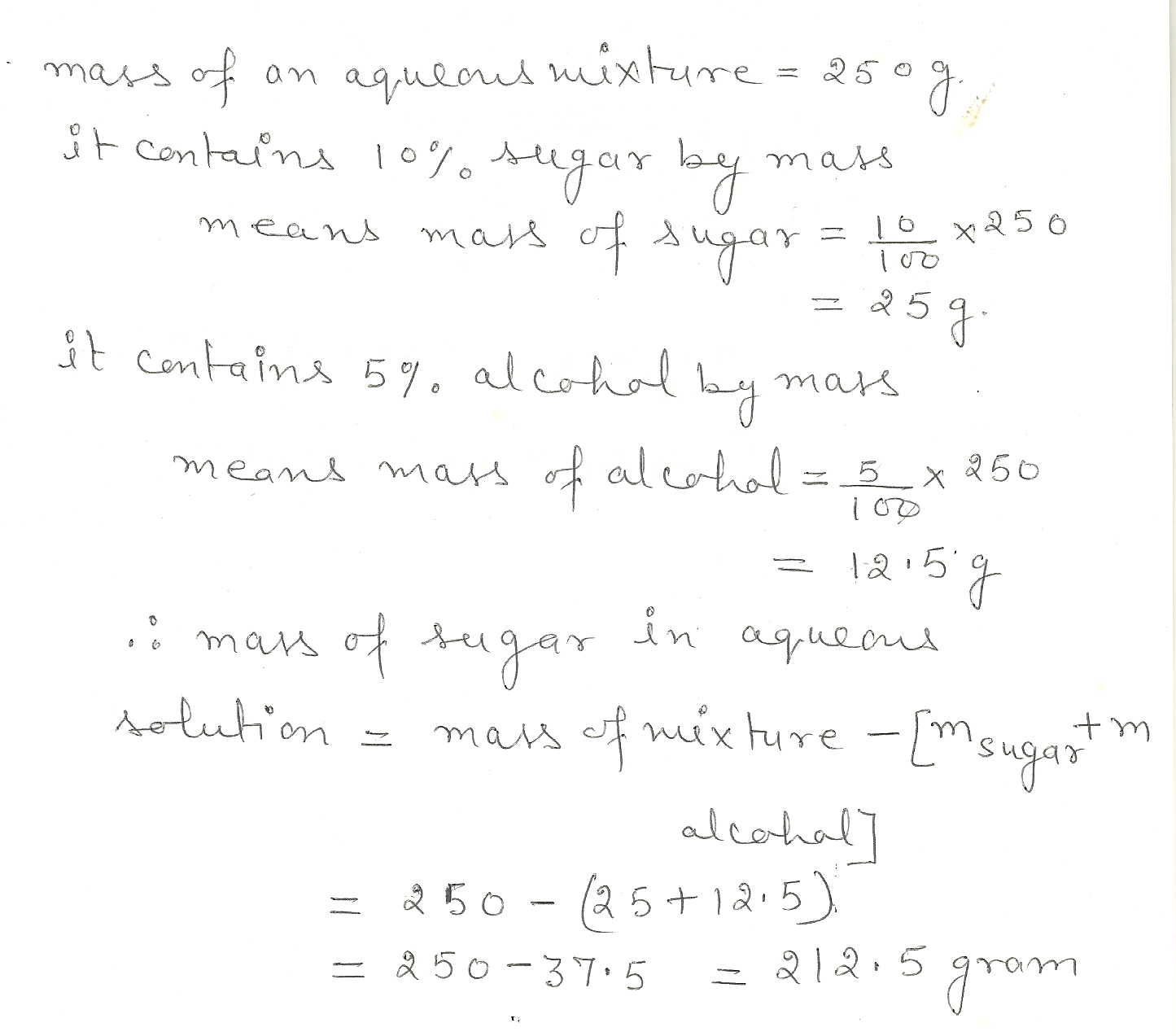4178

Chemistry Inorganic Chemistry Level: Misc Level

Potassium permanganate , KMn04, is produced commercially by oxidising aqueous potassium manganate, K2MnO4, with chlorine gas.The chemical equation is:

2MnO42-(aq)+ CI2(g) +2K+ (aq) -> 2KMn04 (s) +2CI- (aq)

What volume of CI2 (g), measured at STP, is needed to produce 10.0 g of KMn04?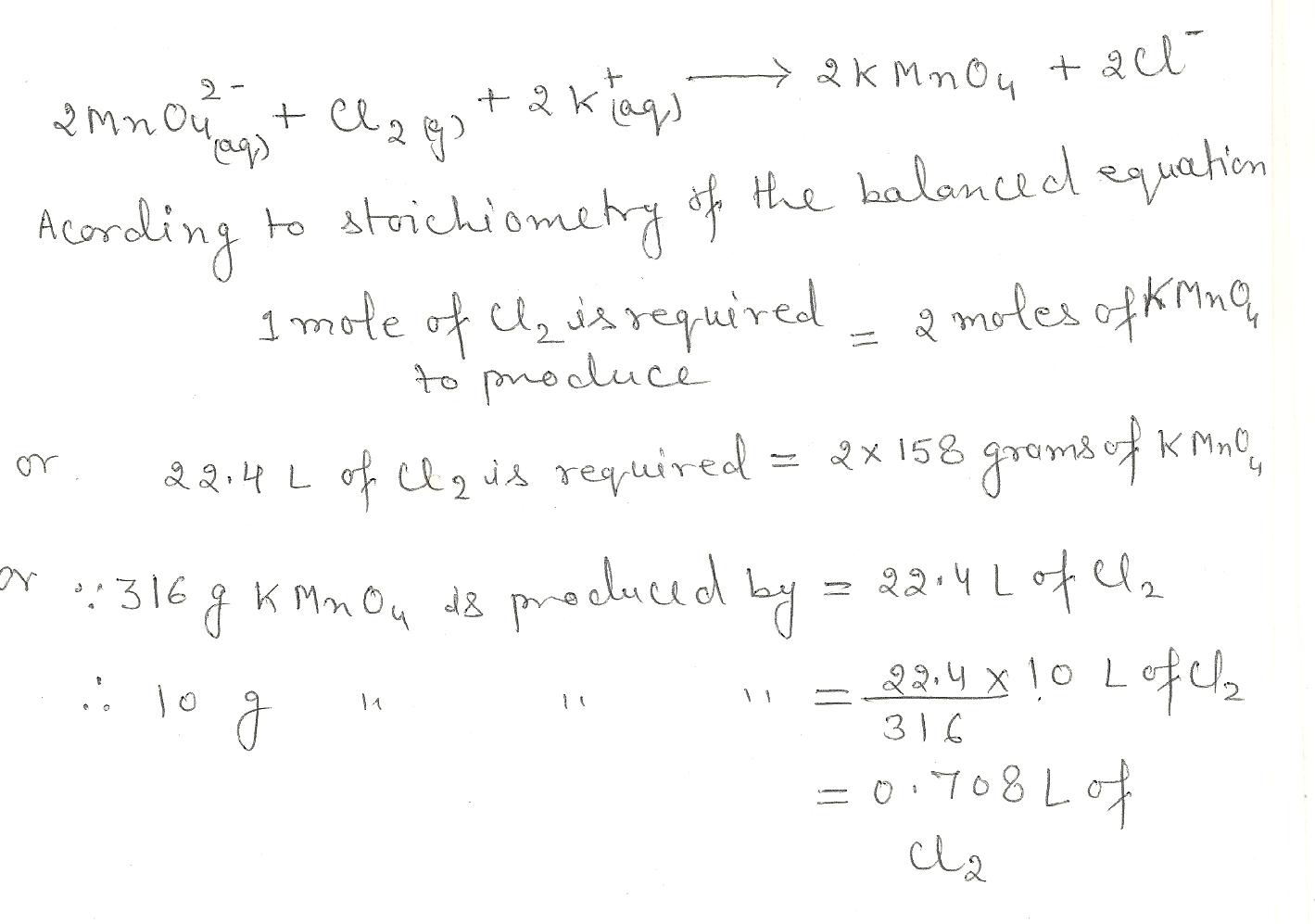4137

Chemistry Inorganic Chemistry Level: Misc Level

(b) what is the pH for the following solution? 0.001 M HNO3 ch.9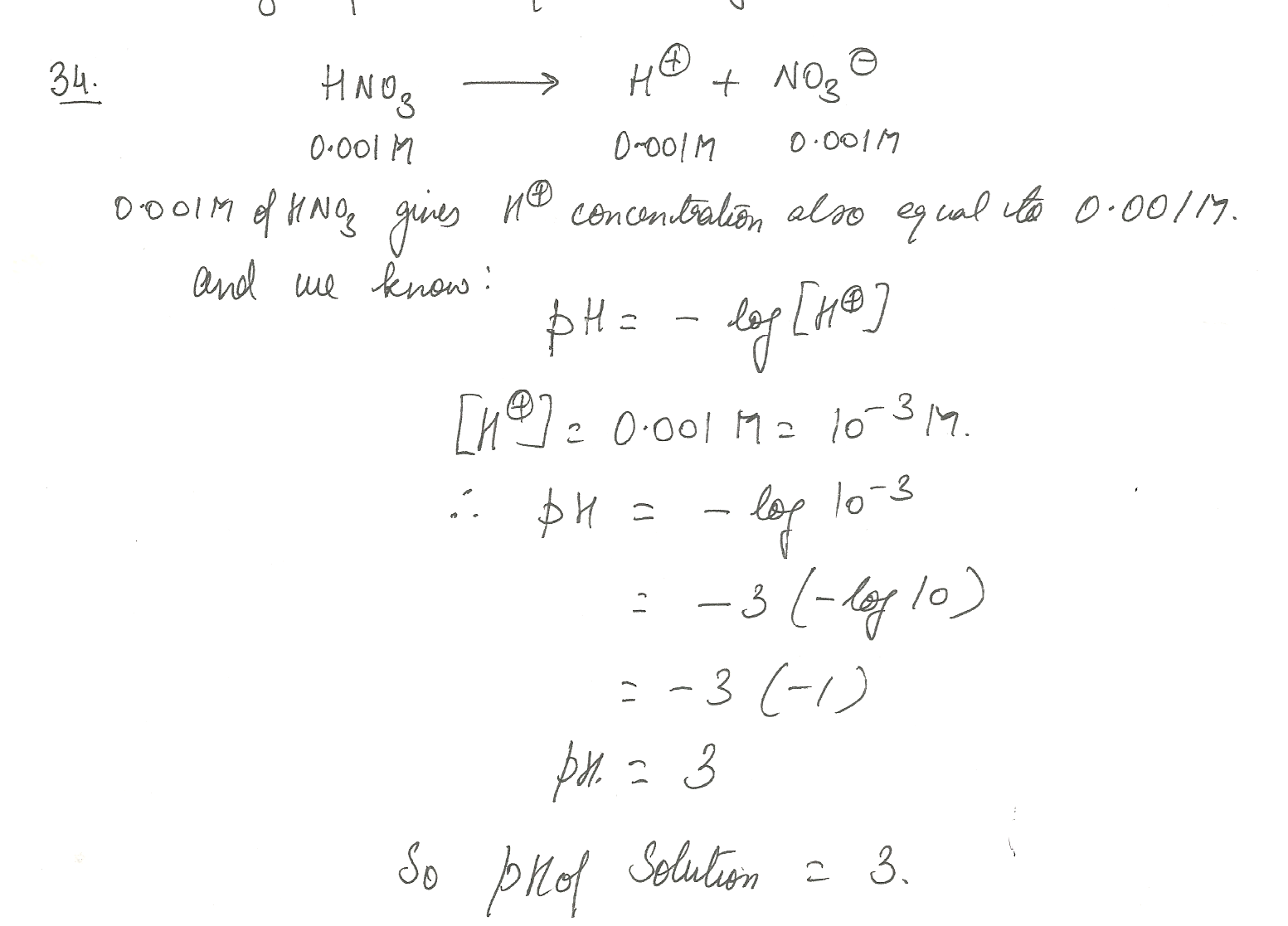4136

Chemistry Inorganic Chemistry Level: Misc Level

How many grams of HBr must be dissolved to make 1.0 L of 2.0 M HBr solution?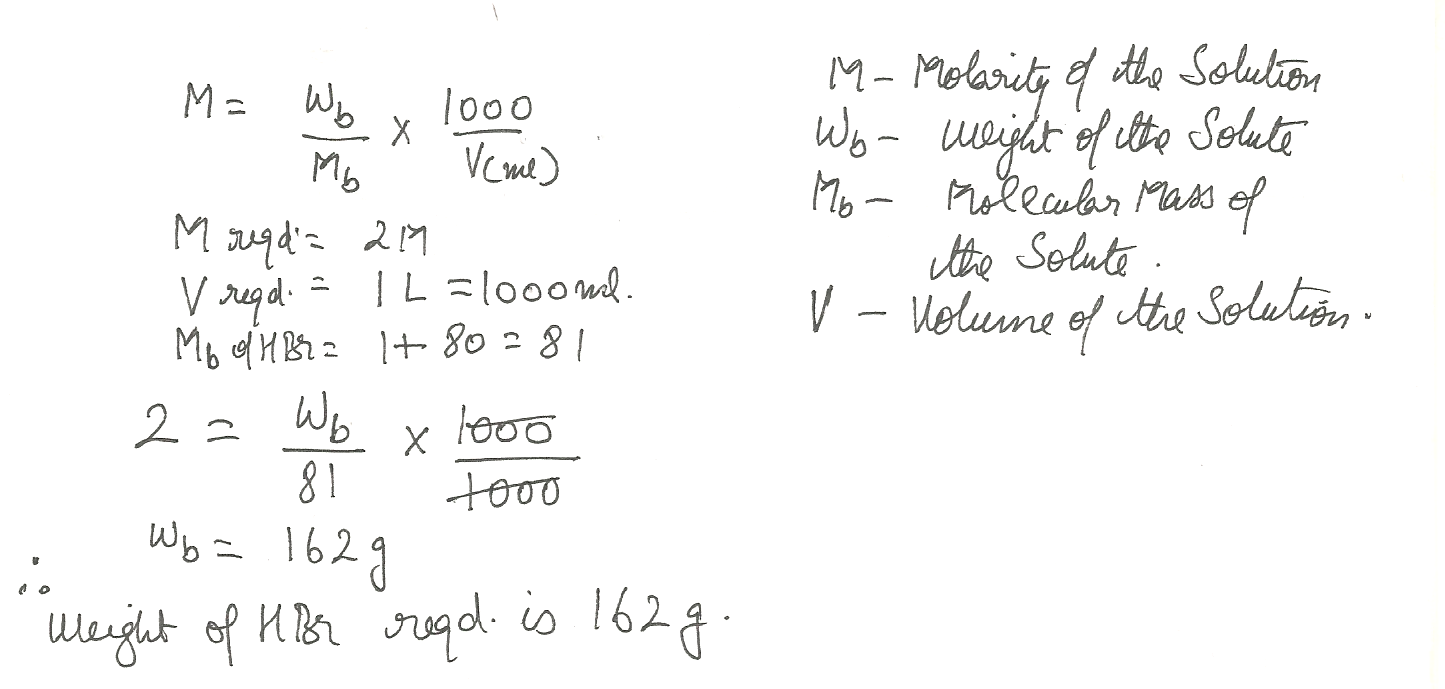Displaying 46-60 of 61 results.